Computational & Technology Resources
an online resource for computational,
engineering & technology publications
Civil-Comp Proceedings
ISSN 1759-3433
CCP: 83
PROCEEDINGS OF THE EIGHTH INTERNATIONAL CONFERENCE ON COMPUTATIONAL STRUCTURES TECHNOLOGY
Edited by: B.H.V. Topping, G. Montero and R. Montenegro
Paper 204

Bias-Specified Robust Design Optimization: An Alternative Approach

G. Steenackers and P. Guillaume

Acoustics & Vibration Research Group, Department of Mechanical Engineering, VUB University Brussels, Belgium

Full Bibliographic Reference for this paper
G. Steenackers, P. Guillaume, "Bias-Specified Robust Design Optimization: An Alternative Approach", in B.H.V. Topping, G. Montero, R. Montenegro, (Editors), "Proceedings of the Eighth International Conference on Computational Structures Technology", Civil-Comp Press, Stirlingshire, UK, Paper 204, 2006. doi:10.4203/ccp.83.204
Keywords: optimization, robust design, regression techniques.

Summary
This paper discusses an existing robust optimization approach, suggested and applied in the work of the authors Shin et al. , and presents an alternative robust optimization approach. Shin et al. suggest an optimization model, using Lagrange multipliers to combine both constraints and slack variables to change the inequality constraints to equality constraints. The cost function used, proposed by the authors, consists of minimizing the variance (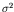) while the extra inequality constraint of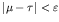is considered and taken into account by using Lagrange multipliers. The optimization strategy is to minimize the variance while the difference between process mean and target value should be situated within (allowable) user-specified interval boundaries. Our proposed algorithm, based on a generalized robust optimization approach, will consist out of a standard Gauss-Newton optimization algorithm  in combination with a mean squared error function as the cost function to be minimized. An alternative robust design optimization approach consists of using a different cost function by minimizing the mean squared error, which taken into account both the variance () and the difference (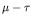) between process mean and target value. Lagrange multipliers are again used in order to take into account the extra inequality constraint.

Let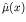and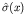represent the fitted response functions for the mean and standard deviation of the design parameter vector X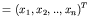. The second-order response functions fitted for the mean and standard deviation are obtained as: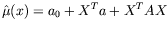(33)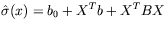(34)

with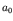and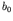regression constants, a and b (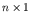)-vectors with constant values and A and B (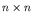)-matrices with constant element values.

The optimization problem is solved by using a numerical regression technique. For solving the generalized least squares problem, one needs to specify three initial design parameter value pairs where the correct value of the updating parameter is situated in between . The least squares problem is solved with the boundary values used as starting values. A regression algorithm is used to calculate the regression coefficients of the interpolation polynomials. The difference in this alternative optimization approach is the fact that the mean squared error is minimized by using a cost function consisting of both the process variance and the difference between process mean and process target. In the optimization approach described in  only the variance was minimized, taking into account the extra inequality constraint that the process mean must be situated in a region around the process target, pre-defined by the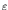-value. As an example, both optimization approaches are illustrated with a numerical test case. The results are explained and discussed.

The generalized robust optimization approach, minimizing the mean squared error of both process mean deviation and variance, will have a mean value closer to the process target value for almost the same variance value, reached with the original robust optimization approach. The reason for this better optimization result is the fact that the generalized optimization approach minimizes the mean square error of () and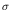instead of only minimizing. Ignoring rounding errors, both robust optimization algorithms give the same results when using the same cost function expression. Also, when using a cost function only containing, the process mean is within the pre-defined target region. Thus, it is not necessary to use Lagrange multipliers in order to take into account the extra inequality constraint. The optimized process mean is situated in the allowable user-defined target region and the inequality constraint is automatically satisfied without the usage of Lagrange multipliers. When considering a cost function, consisting only out of the difference between process mean and bias (), the process bias reaches 0 which is the target when applying optimal design instead of robust design.

References
1
S. Shin, B.R. Cho, "Bias-specified robust design optimization and its analytical solutions", Computers & Industrial Engineering, 48, 129-140, 2005. doi:10.1016/j.cie.2004.07.011
2
J. Berger, "Statistical decision theory", Springer-Verlag, Berlin Heidelberg, 1995.
3
G. Steenackers, P. Guillaume, "Structural design optimization using a regressive finite element updating approach", Proceedings of the 23rd IMAC Conference, Orlando, Fl., 2005.
4
The MathWorks, "Matlab Optimization Toolbox User's Guide", 2004.
5
E. Polak, "Computational Methods in Optimization - A Unified Approach", Mathematics in Science and engineering, 77, Academic Press, 1971.

purchase the full-text of this paper (price £20)